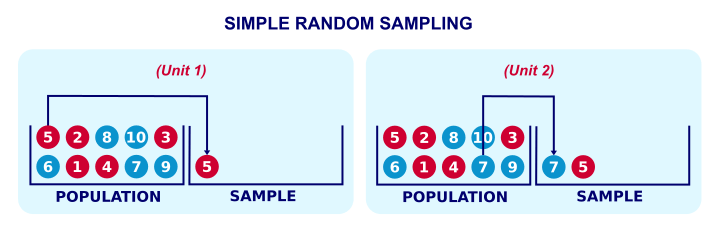# Random sampling Three ways of obtaining a Random Sample

### September 26, 2019

Random Sampling and three ways of obtaining a Random sampleDefinition of 'Random Sampling' Definition: Random sampling is a part of the sampling technique in which each sample has an equal probability of being chosen. A sample chosen randomly is meant to be an unbiased representation of the total population.
Definition: Random sampling is a part of the sampling technique in which each sample has an equal probability of being chosen. A sample chosen randomly is meant to be an unbiased representation of the total population. If for some reasons, the sample does not represent the population, the variation is called a sampling error.

Simple random sampling is a sampling technique where every item in the population has an even chance and likelihood of being selected in the sample. Here the selection of items completely depends on chance or by probability and therefore this sampling technique is also sometimes known as a method of chances.

This process and technique is known as simple random sampling, and should not be confused with systematic random sampling. A simple random sample is a fair sampling technique.

Simple random sampling is a very basic type of sampling method and can easily be a component of a more complex sampling method. The main attribute of this sampling method is that every sample has the same probability of being chosen.

The sample size in this sampling method should ideally be more than a few hundred so that simple random sampling can be applied appropriately. It is sometimes argued that this method is theoretically simple to understand but difficult to practically implement. Working with large sample size isn’t an easy task and it can sometimes be a challenge finding a realistic sampling frame.Simple random sampling methods
The following steps are involved in selecting simple random sampling:
1. A list of all the members of the population is prepared initially and then each member is marked with a specific number ( for example, there are nth members then they will be numbered from 1 to N).
2. From this population, random samples are chosen using two ways: random number tables and random number generator software. A random number generator software is preferred more as the sample numbers can be generated randomly without human interference.

Two approaches aim to minimize any biases in the process of simple random sampling:
Method of lottery

Using the method of the lottery is one of the oldest methods and is a mechanical example of random sampling. In this method, each member of the population has to number systematically and in a consequent manner by writing each number on a separate piece of paper. These pieces of paper are mixed and put into a box and then numbers are drawn out of the box randomly.Advantages of Simple Random Sampling
1. It is a fair method of sampling and if applied appropriately it helps to reduce any bias involved as compared to any other sampling method involved.
2. Since it involves a large sample frame it is usually easy to pick smaller sample size from the existing larger population.
3. The person who is conducting the research doesn’t need to have prior knowledge of the data being collected. One can simply ask a question to gather the researcher need not be a subject expert.
4. This sampling method is a very basic method of collecting the data. There is no technical knowledge required and need basic listening and recording skills.
5. Since the population size is large in this type of sampling method there is no restriction on the sample size that needs to be created. From a larger population, you can get a small sample quite easily.
6. The data collected through this sampling method is well informed, more the samples better are the quality of the data.
Disadvantages of Simple Random Sampling
1. It is a costlier method of sampling as it requires a complete list of all potential respondents to be available beforehand.
2. This sampling method is not suitable for studies involving face-to-face interviews as covering large geographical areas have cost and time constraints.
3. A sample size that is too large is also problematic since every member of the population has an equal chance of selection. The larger population means a larger sample frame. It is difficult to manage a large population.
4. The quality of the data depends on the researcher and his/her perspective. If the researcher is experienced then there are fair chances the quality of data collected is of superior quality. But if the researcher is inexperienced then the data collected may or may not be up to the mark.FB Page

@ELITE_Mv